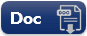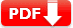# Average Math Practice Test Question Answers: Set 1

Average Math Practice Test Question Answers: This kind of problem-solving math test helps various exam prep. You can download free printable and editable PDF and Doc files for worksheet purposes.

Average Math Practice Test Question Answers: Set 1

 1. Robert obtained 74, 65, 87, 55 and 90marks (out of 100) in Living Environment, Earth Science, Chemistry, Global History, and Geography. What are his average marks ? 🔘 71.5 🔘 69.60 🔘 81.68 🔘 83.54 🔘 NOTA
Answers: E None of the above
 2. A grocer has a sale of $6435 ,$ 6927 , $6855 ,$ 7230 and $6562 for 5 consecutive months. How much sale must he have in the sixth month so that he gets an average sale of$ 6500 ? 🔘 $3568 🔘$3987 🔘 $4991 🔘$5611 🔘 $6147 Show Answers Answers: C$4991
 3. William buys diesel at $7.50 ,$8 and $8.50 per litre for three successive years. What approximately is the average cost per litre of diesel if he spends$4000 each year? 🔘 $6.58 🔘$7.14 🔘 $7.98 🔘$8.12 🔘 $8.41 Show Answers Answers: C$7.98
 4. A family consists of two grandparents, two parents and three grandchildren. The average age of the grandparents is 67 years, that of the parents is 35 years and that of the grandchildren is 6 years. What is the average age of the family in years? 🔘 25.31 🔘 29.65 🔘30.27 🔘 31.71 🔘 34.52
 5. There are two groups “Full House” and “Duck Duck Goose” of a class, consisting of 36 and 44 students respectively. If the average weight of he group “Full House” is 40 kg and that of he group “Duck Duck Goose” is 35 kg, what is the average weight of the whole class? 🔘 35.25 🔘 36.25 🔘 35.50 🔘 36.50 🔘 37.25
 6. The average age of boys in a class is 16.5 years and that of the girls is 15.5 years. What is the average age of the whole class? 🔘 15 🔘 16 🔘 15.9 🔘 Insufficient data 🔘 NOTA
Answers: D. Insufficient data (number of boys and girls are missing)
 7. Average of six numbers is x and average of three of these is y . If the average of remaining three is z , then 🔘 x=2y+2z 🔘 2x=y+z 🔘 x=y+z 🔘 x=(y+z)2 🔘 NOTA
 8. The average monthly income of Lucas and Elijah is $5050. The average monthly income of Elijah and Benjamin is$6250 and the average monthly income of Lucas and Benjamin is $5200. What is the monthly income of Lucas? 🔘$1000 🔘 $2000 🔘$3000 🔘$4000 🔘$6000
 Document Type Download Link Free Editable Doc FileFree Printable PDF File